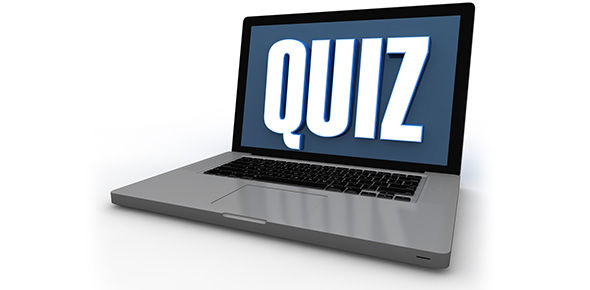# Triangles And 3-dimensional Figures

10 Questions | Total Attempts: 41SettingsA quiz to test knowledge of 3-dimensional figures and to identify different types of triangles. A quiz for 7th grade mathematics.

• 1.
1.  A polyhedron whose base is a polygon and whose faces are triangles that have a common vertex is a
• A.

Cylinder

• B.

Sphere

• C.

Cone

• D.

Pyramid

• 2.
A simple closed surface that is bounded by two congruent circles that lie in parallel planes form a
• A.

Cylinder

• B.

Cone

• C.

Pyramid

• D.

Prism

• 3.
A simple closed surface whose base is a simple closed surface and whose lateral surface slopes up to a vertex is a(n)
• A.

Obtuse triangle

• B.

Cone

• C.

Cylinder

• D.

Sphere

• 4.
All polyhedra that have two parallel bases that are congruent polygons are called
• A.

Apexes

• B.

Acute triangles

• C.

Prisms

• D.

Cones

• 5.
The point formed when the lateral surface of a cone creates a vertex is also known as the apex.
• A.

True

• B.

False

• 6.
A triangle that contains an angle greater than 90 degrees is a(n)
• 7.
A triangle that contains 3 angles that are each less than 90 degrees is a(n)
• 8.
A triangle that contains 1 right angle (90 degree angle) is a(n)
• 9.
A triangle in which all 3 sides have different lengths (no 2 sides have the same length) is a(n)
• 10.
If a triangle's sides are all the same length, it is known as a(n)
Related TopicsBack to top